Study Guide

# Scale Models & Similarity

## Scale Models & Similarity

### Scale Models

Mini-Me is a clone, identical in every way to Dr. Evil, except that he'shis size. The ratioor 1:8 means that for every 1 inch of Mini-Me, there are 8 inches of pure Dr. Evil. This ratio can also be called a scale factor. A scale factor can be used for any two similar figures.

There are a lot of "mini-me's" (the technical term being scale models) in the world. Architects make a mini-building before they make the real thing. Car designers make a mini-car before they make the real thing. Evil scientists make a mini-death ray before they make the real thing. Each of these minis has a scale factor, or a ratio telling how big the mini is compared to how big the real thing will be. Scale factors can be found on blueprints for buildings, maps of the world, and instructions to build a tank, but don't tell Dr. Evil. We don't want him getting any big ideas.

### Sample Problem

If a model car has a scale factor of 1:25 and the model is 3 inches wide, how wide would the actual car be in feet?

We solve scale factor problems with proportions. The scale factor 1:25 means that for every 1 inch (or foot or cm or any measurement) of the model, there will be 25 inches (or any same measurement) of the real car. The numerator is a measurement from the model, and the denominator is a measurement from the real car.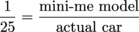Let's set up a proportion. The scale factor is our first ratio. The second ratio will have real measurements in it: the measurements of the model in the numerator and the measurement of the real car in the denominator. We'll use the variable x for the real car's width.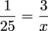We solve by multiplying both sides by 25x, because our denominators are 25 and x. Then we cancel whatever we can.We got x = 75 inches, so the actual car will be 75 inches wide. But wait, there's more. If you act now, you too can own a car measured in feet rather than inches (since the problem asked us for the car's width in feet). For the measurement whizzes out there, we can divide 75 inches by 12, since there are 12 inches in a foot, and get 6.25 feet. But if that sounds like mumbo-jumbo, proportions come to our rescue once again.

To convert 75 inches into feet, we can create two ratios like this: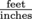. The first ratio tells how many inches are in 1 foot, and the second ratio tells the measurements of the car. Hold up; we don't know how many feet long our car is. Yup. So we use x.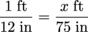This time, we'll multiply both sides of our proportion by (12)(75) to get rid of those denominators.Dividing both sides by 12 we get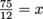, or x = 6.25 feet.

### Similar Figures

Sometimes we're given two similar figures with measurements, but we're not given the scale factor. Luckily, we can use a corresponding measurement from each figure to create a scale factor and then use that scale factor to find any other missing measurements.

### Sample Problem

Dr. Evil was given two similar models of an Egyptian pyramid he wants to steal. The smaller model has a base length of 4 inches and a height of 10 inches. The larger model has a base length of 18 inches. Dr. Evil wants to know the height of the larger model to make sure it fits in his travel bag. He makes Mini-Me do the math.

Here's a picture of each pyramid's cross-section.Mini-Me sets up a ratio of measurements for the small pyramid (length:height) and a ratio of measurements for the large pyramid (also length:height), and then he solves for x.Dividing both sides by 4, he gets: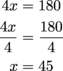He's got it: x = 45 inches.

The height of the larger pyramid is 45 inches, or just under 4 feet. Probably too large to take on Dr. Evil's trip, which is fine since he's planning on bringing the real pyramid home with him.